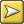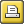﻿ plot dispersion value
 Plot dispersion valueThe point of it

A dispersion value is the degree to which a set of values are uniformly spread. Think of rainfall in the UK -- generally fairly uniformly spread throughout the year. Compare with countries which have a rainy season.

In linguistic terms, one might wish to know how the occurrences of a word like skull are distributed in Hamlet, and WordSmith has shown this in plot form since version 1. The dispersion value statistic gives mathematical support to this and makes comparisons easier.

How it is calculated

The plot dispersion calculated in KeyWords and Concord dispersion plots uses the first of the 3 formulae supplied in Oakes (1998: 190-191), which he reports as having been evaluated as the most reliable.

Like the ruler, it divides the plot into 8 segments for this.

It ranges from 0 to 1, with 0.9 or 1 suggesting very uniform dispersion and 0 or 0.1suggesting "burstiness" (Katz, 1996)